Next:3.5.1 Division MethodUp:3. DictionariesPrevious:3.4.3 Another Example:

# 3.5 Hashing Functions

What is a good hash function?
• Should satisfy the simple uniform hashing property.

•

Let U = universe of keys

Let the hash values be 0, 1, . . . , m-1

Let us assume that each key is drawn independently from U according to a probability distribution P. i.e., for k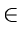P(k) =  Probability that  k  is drawn
Then simple uniform hashing requires that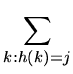P(k) =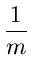for each  j = 0, 1,..., m - 1
that is, each bucket is equally likely to be occupied.

• Example of a hash function that satisfies simple uniform hashing property:

•

Suppose the keys are known to be random real numbers k independently and uniformly distributed in the range [0,1).

h(k) =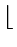km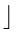satisfies the simple uniform hashing property.

Qualitative information about P is often useful in the design process. For example, consider a compiler's symbol table in which the keys are arbitrary character strings representing identifiers in a program. It is common for closely related symbols, say pt, pts, ptt, to appear in the same program. A good hash function would minimize the chance that such variants hash to the same slot.

• A common approach is to derive a hash value in a way that is expected to be independent of any patterns that might exist in the data.
• The division method computes the hash value as the remainder when the key is divided by a prime number. Unless that prime is somehow related to patterns in the distribution P, this method gives good results.Next:3.5.1 Division MethodUp:3. DictionariesPrevious:3.4.3 Another Example:
eEL,CSA_Dept,IISc,Bangalore